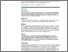# The upper limits of risk ratios and recommendations for reporting risk ratios, odds ratios, and rate ratios

Chao, Yi-Sheng, Wu, Chao-Jung, , Huang, Shih-Yu, Wu, Hsing-Chien, Hsu, Hui-Ting, Cheng, Yen-Po, Lai, Yi-Chun and Chen, Wei-Chih (2023) The upper limits of risk ratios and recommendations for reporting risk ratios, odds ratios, and rate ratios. Cureus Journal of Medical Sciences, 15 (4):e37799. pp. 1-22. ISSN 2168-8184 (doi:https://doi.org/10.7759/cureus.37799)Preview
PDF (Published manuscript VoR)
41783_PO_The_upper_limits_of_risk_ratios_and_recommendations_for_reporting_risk_ratios.pdf - Published Version

## Abstract

Background
Relative measures, including risk ratios (RRs) and odds ratios (ORs), are reported in many epidemiological studies. RRs represent how many times a condition is likely to develop when exposed to a risk factor. The upper limit of RRs is the multiplicative inverse of the baseline incidence. Ignoring the upper limits of RRs can lead to reporting exaggerated relative effect sizes.
Objectives
This study aims to demonstrate the importance of such upper limits for effect size reporting via equations, examples, and simulations and provide recommendations for the reporting of relative measures.
Methods
Equations to calculate RRs and their 95% confidence intervals (CIs) were listed. We performed simulations with 10,000 simulated subjects and three population variables: proportions at risk (0.05, 0.1, 0.3, 0.5, and 0.8), baseline incidence (0.05, 0.1, 0.3, 0.5, and 0.8), and RRs (0.5, 1.0, 5.0, 10.0, and 25.0). Subjects were randomly assigned with a risk based on the set of proportions-at-risk values. A disease occurred based on the baseline incidence among those not at risk. The incidence of those at risk was the product of the baseline incidence and the RRs. The 95% CIs of RRs were calculated according to Altman.
Results
The calculation of RR 95% CIs is not connected to the RR upper limits in equations. The RRs in the simulated populations at risk could reach the upper limits of RRs: multiplicative inverse of the baseline incidence. The upper limits to the derived RRs were around 1.25, 2, 3.3, 10, and 20, when the assumed baseline incidence rates were 0.8, 0.5, 0.3, 0.2, and 0.05, respectively. We demonstrated five scenarios in which the RR 95% CIs might exceed the upper limits.
Conclusions
Statistical significance does not imply the RR 95% CIs not exceeding the upper limits of RRs. When reporting RRs or ORs, the RR upper limits should be assessed. The rate ratio is also subject to a similar upper limit. In the literature, ORs tend to overestimate effect sizes. It is recommended to correct ORs that aim to approximate RRs assuming outcomes are rare. A reporting guide for relative measures, RRs, ORs, and rate ratios, is provided. Researchers are recommended to report whether the 95% CIs of relative measures, RRs, ORs, and rate ratios, overlap with the range of upper limits and discuss whether the relative measure estimates may exceed the upper limits.

Item Type: Article 95% confidence interval; odds ratios; upper limits; rate ratios; relative measures; risk ratios Q Science > QA MathematicsQ Science > QR Microbiology > QR355 VirologyR Medicine > RA Public aspects of medicine > RA0421 Public health. Hygiene. Preventive Medicine Faculty of Engineering & ScienceFaculty of Engineering & Science > Natural Resources InstituteFaculty of Engineering & Science > Natural Resources Institute > Livelihoods & Institutions Department 25 Apr 2023 08:41 http://gala.gre.ac.uk/id/eprint/41783View Item